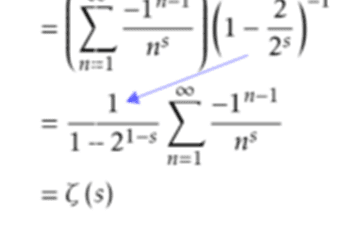# Taming a Divergent Series -- But how does it work?

• I
• Swamp Thing

#### Swamp Thing

A convergent version ( i.e. convergent in the critical strip) of the traditional series for the Riemann Zeta is derived in the video linked at the bottom. It gives the correct numerical values (at least along the critical line, where I tried it out).

But although it works numerically, I'm wondering whether it is rigorously correct. That is, does the derivation follow the strict rules about how series are to be manipulated? (I am not an expert on those rules, so...)

For example the derivation replaces (in effect)
$$\sum_{n=1}^\infty 1/n^s$$
with
$$\sum_{n=1}^\infty \{ {1/(2n-1)}^s + 1/(2n)^s\}$$

This replacement, though invalid for a finite sum, may well be valid when summing to infinity. However, I'm not sure if the second version above can then be merged with another sum (which is what happens around 07:02 in the video). It seems to me that if we write the sums with independent indices M1 and M2, we would get an error if we then tried to set M1 = M2 = M -- at least for finite M. Given this fact, running the M up to infinity should also be problematic.

But again, it does work numerically, so this trick must be justifiable. So what is going on and how does it all work?

Last edited:
•Delta2
Is that even an analytic continuation?

$$\sum_{n=1}^{\infty } \left(\dfrac{1}{(2n-1)^2}+\dfrac{1}{2n^2}\right)=\dfrac{5}{24}\pi^2\neq \dfrac{4}{24}\pi^2=\zeta(2)$$
and your series isn't even defined for ##n=0.##

fresh_42 said:
$$\sum_{n=1}^{\infty } \left(\dfrac{1}{(2n-1)^2}+\dfrac{1}{2n^2}\right)=\dfrac{5}{24}\pi^2\neq \dfrac{4}{24}\pi^2=\zeta(2)$$
and your series isn't even defined for ##n=0.##

Maybe it's 'my bad' ... I should clarify that the substitution quoted in my original post is only an intermediate step. After doing that, he subtracts another scaled copy of the original series.

The final formula derived in the video is this:And it does work.
Code:
fnSumz[s_,
N_] := (1/(1 - 2^(1 - s)))  Sum[((-1)^(n - 1))/(n^s), {n, 1, N}]
vv = 0.5 + I 14.1347;
Zeta[vv]
fnSumz[vv, Infinity]

3.13536*10^-6 -0.0000196934 I
3.13536*10^-6 -0.0000196934 I

Swamp Thing said:
A convergent version ( i.e. convergent in the critical strip) of the traditional series for the Riemann Zeta is derived in the video linked at the bottom. It gives the correct numerical values (at least along the critical line, where I tried it out).

But although it works numerically, I'm wondering whether it is rigorously correct. That is, does the derivation follow the strict rules about how series are to be manipulated? (I am not an expert on those rules, so...)

For example the derivation replaces (in effect)
$$\sum_{n=0}^\infty 1/n^s$$
with
$$\sum_{n=0}^\infty \{ {1/(2n-1)}^s + 1/2n^s\}$$

The lower limit should be 1 in each case.

Assuming the original series is absolutely convergent, we can reorder the terms as we like without affecting the limit, in this case by taking a term for odd $n = 2m - 1$ followed by the next term for even $n = 2m$ together; and $n$ is just a dummy variable so $$\sum_{n=1}^\infty a_n = \sum_{m=1}^\infty (a_{2m-1} + a_{2m}) = \sum_{n=1}^\infty (a_{2n-1} + a_{2n}).$$ (This does also work for finite series, provided the original upper limit $N = 2M$ is even and is replaced by $M$ in the new expression.)

pasmith said:
The lower limit should be 1 in each case.
Oops! I'll correct that.

fresh_42 said:
$$\sum_{n=1}^{\infty } \left(\dfrac{1}{(2n-1)^2}+\dfrac{1}{2n^2}\right)=\dfrac{5}{24}\pi^2\neq \dfrac{4}{24}\pi^2=\zeta(2)$$
and your series isn't even defined for ##n=0.##
But, doesn't it go from ##0## to ##\infty##? And, as @drmalawi points out, it's an issue of determining the ( possibility of) an Analytic Continuation beyond the original disk of convergence. There are cases where there are barriers preventing the continuation beyond the boundary of a disk.

•malawi_glenn
WWGD said:
But, doesn't it go from ##0## to ##\infty##?
No. ##n=0## isn't defined. It is impossible to start at ##0.##

fresh_42 said:
No. ##n=0## isn't defined. It is impossible to start at ##0.##
Edit:Sorry, meant to say from ##1## to##\infty##

Last edited:
WWGD said:
Sorry, meant to say from ##0## tob##\infty##
fresh_42 said:
No. ##n=0## isn't defined. It is impossible to start at ##0.##

Brain fart , from ##1## to ##\infty##

...and the 2nd summand is meant to be ##1/(2n)^s##, right?

I am still curious about that proposed analytic continuation, could someone elaborate on that?

vanhees71 said:
...and the 2nd summand is meant to be ##1/(2n)^s##, right?
True.

Corrected in OP. (Also corrected the summing from zero, which should be from 1).

Swamp Thing said:
True.

Corrected in OP. (Also corrected the summing from zero, which should be from 1).
••